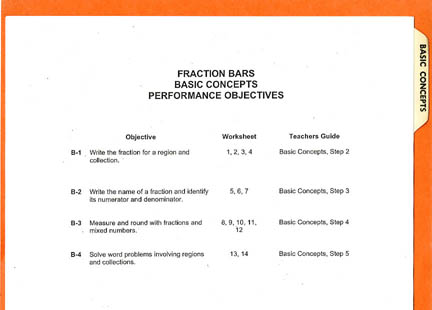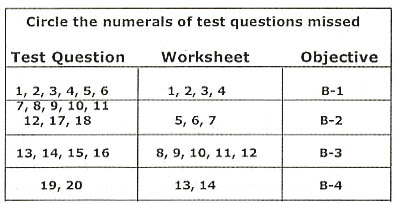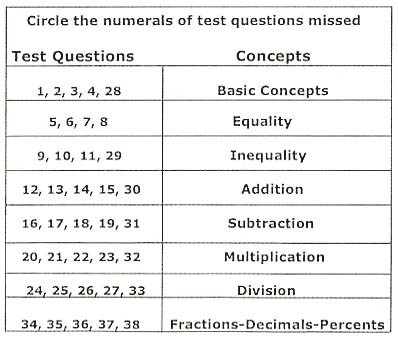Home How to Use Grades 5-8 Teacher Resource Package . Purpose The purpose of the Grades 5-8 Fraction Bars Teacher Resource Package is to provide students with an opportunity to practice concepts and skills obtained from the games and activities in the Grades 5-8 Teacher’s Guide and to provide assessment of the performance objectives on the eight tabbed dividers of the resource package. . Tabbed Dividers The eight tabbed dividers in this package match the eight main sections or topics in the Grades 5-8 Fraction Bars Teacher’s Guide. The Basic Concepts divider is shown here. The dividers should be arranged in the following order: Basic Concepts, Equality, Inequality, Addition, Subtraction, Multiplication, Division, and Fractions-Decimals-Percents. One practice is to put the Progress Charts in front of the dividers and the Achievement Test and answers behind the last divider. Each section contains the following: . . . Reproducible worksheets to reinforce the concepts presented in the Teacher’s Guide . . . Answers for the worksheets . . . Performance Objectives . . . A reproducible Performance Test that evaluates concepts presented in the section . . . Answers for the Performance TestWorksheets The worksheets involve the context of the games and activities in the Teacher’s Guide but do not require materials such as game mats, Fraction Bars, or the Fraction Playing Cards. Progress Charts The Student Progress Chart and Class Progress Chart are at the front of the Teacher Resource Package. The Student Progress Chart enables both the teacher and student to follow progress through the worksheets. Completed worksheets can be denoted by circling the worksheet-page numbers and recording the dates. The Class Progress Chart can be used to keep a record of all students as they complete each of the eight sections. For each section (topic) there is a space to record the date and/or the number of any objectives for which the student needs further work. Performance Tests A table on each Performance Test relates the test questions to the objectives and worksheets of the section. The following table is from the Basic Concepts Performance Test. By circling the questions missed, the teacher can tell which objectives were not accomplished and which worksheets need to be redone. An answer key is included for each performance test.Achievement Test The following table is from the Achievement Test. It relates the questions on this test to the appropriate section or topic. By circling the questions missed, this table can be used to analyze student achievement for each topic and/or determine each student's placement within the Fraction Bars Program. An answer key for this test is included.